# Home

 Stochastic Nature of Quanta-II 22nd October 2016 "The principle of Least Action is this: Of all the different sets of paths along which a conservative system may be guided to move from one configuration to another, with the sum of its potential and kinetic energies equal to a given constant, that one for which the action is the least is such that the system will require only to be started with the proper velocities, to move along it unguided." - Lord Kelvin and P. G. Tait, Treatise on Natural Philosophy, 1912. Note: The path of the least action is the one which is measured by ObsC (v/c ~ 1), the observer with maximum capacity. This observer defines e-v system and 1AB in our discussion. Continuing on, we shall discuss the relationship between the wave-function and the discrete measurement space or j-space.  We know that j-space is a dynamic space, i.e. observers or objects can not be completely still neither can they have infinite velocity.  We need to find a quantity which characterizes the path taken between two points A and B (problem of measurements).  Let us call this quantity Action represented by S.  Please note that the path AB exists on the unit-point sphere SU.Further we assume that the system is conservative, which means that the same path can be measured repeatedly without any loss of information.  In j-space where every single measurement is unique, we can not have a truly conservative system unless we assume VT-symmetry, i.e. we must define the origin in the j-space first.  We also have the information contained within the path AB, measurement of which will require certain amount of resources which are essentially the sum of Kinetic (T) and Potential (V) Energies.  We can define a quantity H s.t. H = T+V.  We call H the Hamiltonian of the path AB.  We can correlate the Hamiltonian H and the Action S for the path AB, using Hamilton-Jacobi Equation: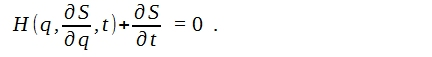If action is independent of time i.e. ∂S/∂t = constant, then we can write the reduced form of Hamilton-Jacobi Equation as: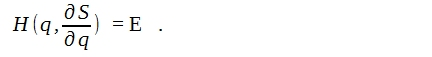The reduced form of Hamilton-Jacobi Equation, is used by Schrödinger to derive the wave equation.  ψ is known as the wave-function.  The time independence of Action S is required so that H can be linearly solved in terms of q's.  The action S now can be written as*: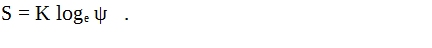In above equation, K is a measurement-metric dependent constant with dimensions of Action S.  The reduced form of Hamilton-Jacobi equation can then be written as :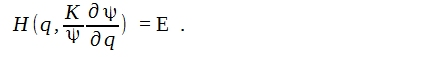Ignoring the relativistic variation in mass, above equation is written as the familiar Schrödinger equation and the constant K is h/2π.  We need to understand the equation S = K logeψ intuitively.     Before we do that however, we must digress a little and discuss the concept of information, in terms of entropy first.  Basically more the information, less the entropy (W) or the disorder (Ω) in the system.  This is the reason, the Information is also known as negative-entropy or Negentropy.  We can define, the Information corresponding to the path AB as measured by the macroscopic observer ObsM, as: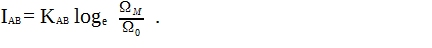The parameters Ω represent number of measurements required to arrive at point B from point A.  For an observer who travels the path AB with one single measurement (i.e. extracting all the available information with a single measurement Ω0), the entropy of the measurement-metric employed by this observer, will be zero since K'loge(1) = 0.  In our case Obsc is the zero-entropy observer.  Another observer ObsM may make a very large number of measurements ΩM, while traveling the same path AB.  Obviously, the entropy of the measurement-metric of the observer ObsM will be very high.  We can take the difference in respective entropies and determine the information IAB contained in path AB.  This information can be translated in to the resources (~energy) needed by ObsM to arrive at point B from A, while extracting all the information contained within the path AB.     Let us now consider the equation S = K logeψ.  Using the analogy above, let us write it for the path AB as follows: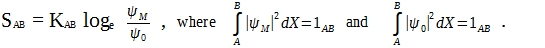SAB - Action corresponding to the path AB. KAB - A constant with the unit of action, corresponding to the path AB. We will discuss this very important constant in detail later. ψM - The measurements made by ObsM while traveling the path AB.  (This will be represented by a Gaussian or one of the stable probability distributions.) ψ0 - The measurements made by Obsc while traveling the path AB. It is essentially a δ-function since Obsc is a maximum capacity observer for the path AB.  1AB - The definition of 1 (~complete information content), for the path AB.  The definition of  1AB, will be based on the measurement made by Obsc.     We are interested in ψM. It represents a small fraction of sample measured by ObsM from an infinitely large population, which is estimated by a probability distribution hence the stochastic nature of ψM.  The nature of the distribution would represent the type of problem being estimated.  These measurements are then compared with the zero-entropy measurement.  An illustrated representation is provided below: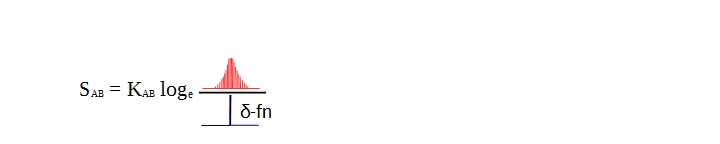What is being said here is that, the Action is being estimated for the path AB on the Unit-point sphere SU, by an observer who within a limited time interval can measure only an infinitesimally small number of samples out of a infinitely large sample space.  We note the value of Action is different for observers of different capacities.  For example the Action has a certain value for the observer Obsc who can measure the information content of the path AB in single measurement and this value is very low.  At the same time for ObsM measuring the same information content, the action value will be very high, as the observer capacity is fairly limited.  The observer ObsM will require lot more resources to move along the path AB unguided.     Finally some remarks about KAB, although we will discuss its significance in the information space later on.  The coefficient KAB is equal to the Boltzmann constant kB in lower information space i.e. thermodynamical system.  But in the discrete measurement space KAB has much broader interpretation.  It's value is based on the measurement metric of the observer ObsC.  If we could calculate the total number of states in universe as measured by ObsM, then this number will represent the number of measurements.  Multiplying the number of states with the Planck constant h, will provide an estimate of resources required to measure the universe by ObsM.  Same measurement will be completed by ObsC by using the resource value equal to h only.  Furthermore since the ObsM has much lower capacity, it can not measure value below h.  Hence the value of Action SAB for ObsC will be an integral multiple of h **.     For a macroscopic observer ObsM, the constant KAB will represent different physical constants corresponding to Action S,  for different paths being measured i.e. different information spaces and subsequently different nature of corresponding disorders ΩM's.  The quantity ΩM represents macro-states in thermodynamic system and quantum-states for a quantum system.  For a lower information space (v/c << 1) KAB will be the Boltzmann physical constant kB (J.K-1), whereas for higher information space (v/c ~ 1) KAB will be the Planck universal constant h (J.s).     Finally, does Quantum Mechanics describe the physical reality?  The physical reality is based on the observer's measurements.  QM provides a powerful method to estimate the physical reality as accurately as possible and it is reflected in the relation ∫|ΨM |2 dX = 1AB.  Perhaps we should ask if there was more to the reality than the physical reality as the observer ObsM perceives it, based on the locality condition?  And if that was the case, how should we describe it? _______________________ * E. Schrödinger, "Quantization as a problem of proper values," Annalen der Physik (4), Vol. 79, 1926.  An English translation is available under the title "Collected papers on Wave-Mechanics" published by Blackie and Son Limited. ** We have discussed this issue at a conceptual level earlier when we introduced ĥ, however we had not explained the correlation with KAB.Previous Blogs:   EPR Paradox-I  Nutshell-2015 Chiral Symmetry Sigma-z and I Spin Matrices Rationale behind Irrational Numbers The Ubiquitous z-Axis Majorana ZFC Axioms Set Theory Nutshell-2014 Knots in j-Space Supercolliders Force Riemann Hypothesis Andromeda Nebula Infinite Fulcrum Cauchy and Gaussian Distributions Discrete Space, b-Field & Lower Mass Bound Incompleteness II The Supersymmetry The Cat in Box The Initial State and Symmetries Incompleteness I Discrete Measurement Space The Frog in Well Visual Complex Analysis The Einstein Theory of Relativity

Best viewed with Internet Explorer